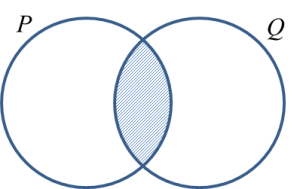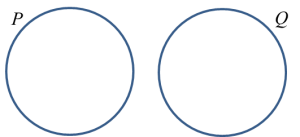# 4.3a Intersection of Sets

4.3a Intersection of Sets

1. The intersection of set P and set Q, denoted by $P\cap Q$  is the set consisting of all elements common to set P and set Q.

2.
The intersection of set P, set Q and set R, denoted by $P\cap Q\cap R$  is
the set consisting of all elements common to set P, set Q and set R.

3.
Represent the intersection of sets using Venn diagrams.

(a) P ∩ Q(b) Q P , then P Q = Q$\begin{array}{l}\end{array}$
(c) P Q = , There is no intersection between set P and set Q .(d) P ∩ Q ∩ RExample 1:
Given that A= {3, 4, 5, 6, 7}, B = {4, 5, 7, 8, 9, 12} and C = {3, 5, 7, 8, 9, 10}.
(a) Find ABC.
(b) Draw a Venn diagram to represent ABC.

Solution:
(a)  ABC= {5, 7}

(b)4. The complement of the intersection of two sets, P and Q, represented by (PQ)’, is a set that consists of all the elements of the universal set, ξ, but not the elements of PQ.

5. The complement of set (PQ)’ is represented by the shaded region as shown in the Venn diagram.